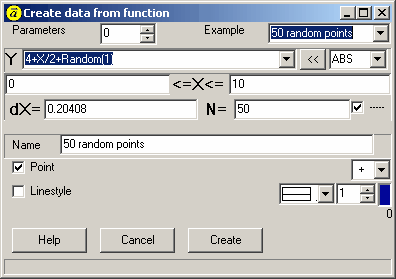50 random pointsPrevious  Top  Next

 · Open the dialog to create a dataset with a function.
2D-Data - > right mouse button - > Add Series- > From function
 · top right you can select different examples.
 · Select the example "50 random points".
 · The X-values consist of 50 values between 0 and 10 with a distance of 0.20408.
 · The X-values are selected in a way that the points lie along the straight line y=4+X/2.
 · In order to have for later evaluations random values, still to each y-value a random value between 0 and 1 is added. Random(1)
 · You can also describe, how the data points should be represented, (point and line type)
 · The dataset gets the name "50 random points"
 · Press now "Create"